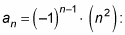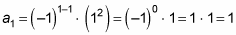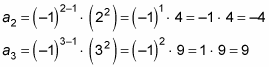##### Math Word Problems For DummiesIf you're given a few terms of a sequence, you can often use these terms to find the general formula for the sequence. If you're given the general formula (complete with n as the variable), you can find the nth term of the sequence by plugging in the position number for n.

The general formula for any sequence involves the letter n, which is the position of the term in the sequence (the first term would be n = 1, and the 20th term would be n = 20), as well as the rule to find each term. You can find any term of a sequence by plugging n into the general formula, which gives you specific instructions on what to do with this value n.

Unless otherwise noted, the first term of any sequence {an} begins with n = 1. The next n always goes up by 1.

For example, you can use the formula to find the first three terms of1. Find a1 first by plugging in 1 wherever you see n.2. Continue plugging in consecutive integers for n.

This process gives you terms two and three: